WHCSRL 技术网

# ID3决策树算法及其Python实现

## 一.理论基础

1. 纯度（purity）

2. 信息熵（information entropy）

``````             Ent(D) = -∑k=1 pk·log2 pk    （约定若p=0，则log2 p=0）

显然，Ent(D)值越小，D的纯度越高。因为0<=pk<= 1,故log2 pk<=0，Ent(D)>=0. 极限情况下，考虑D中样本同属于同一类，则此时的Ent(D)值为0（取到最小值）。当D中样本都分别属于不同类别时，Ent(D)取到最大值log2 |y|.
```123```

3. 信息增益（information gain）

``````           Gain(D,a) = Ent(D)-∑v=1 |Dv|/|D|·Ent(Dv)
```1```

4. 增益率(gain ratio)

``````           Gain_ratio(D,a) = Gain(D,a)/IV(a)
```1```

``````           IV(a) = -∑v=1 |Dv|/|D|·log2 |Dv|/|D|
```1```

``````  事实上，增益率准则对可取值数目较少的属性有所偏好，C4.5算法并不是直接使用增益率准则，而是先从候选划分属性中找出信息增益高于平均水平的属性，再从中选择增益率最高的。
```1```

5. 基尼指数(Gini index)
CART决策树算法使用基尼指数来选择划分属性，基尼指数定义为：

``````          Gini(D) = ∑k=1 ∑k'≠1 pk·pk' = 1- ∑k=1  pk·pk

可以这样理解基尼指数：从数据集D中随机抽取两个样本，其类别标记不一致的概率。Gini(D)越小，纯度越高。

属性a的基尼指数定义：

Gain_index(D,a) = ∑v=1 |Dv|/|D|·Gini(Dv)

使用基尼指数选择最优划分属性，即选择使得划分后基尼指数最小的属性作为最优划分属性。
```123456789```

## 上传数据集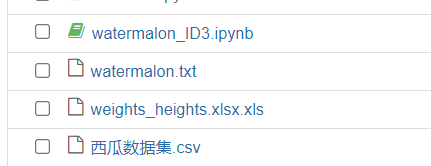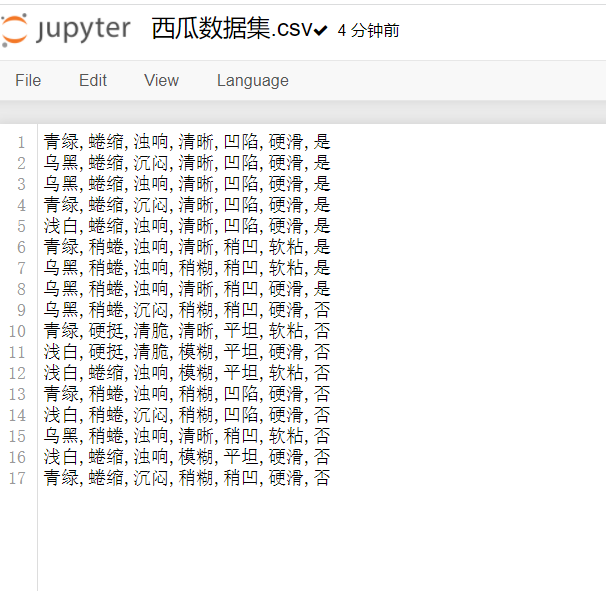## 具体代码

``````import numpy as np
import pandas as pd
import sklearn.tree as st
import math
import matplotlib
import os
import matplotlib.pyplot as plt

data

def calcEntropy(dataSet):
mD = len(dataSet)
dataLabelList = [x[-1] for x in dataSet]
dataLabelSet = set(dataLabelList)
ent = 0
for label in dataLabelSet:
mDv = dataLabelList.count(label)
prop = float(mDv) / mD
ent = ent - prop * np.math.log(prop, 2)

return ent

# index - 要拆分的特征的下标
# feature - 要拆分的特征
# 返回值 - dataSet中index所在特征为feature，且去掉index一列的集合
def splitDataSet(dataSet, index, feature):
splitedDataSet = []
mD = len(dataSet)
for data in dataSet:
if(data[index] == feature):
sliceTmp = data[:index]
sliceTmp.extend(data[index + 1:])
splitedDataSet.append(sliceTmp)
return splitedDataSet
# 返回值 - 最好的特征的下标
def chooseBestFeature(dataSet):
entD = calcEntropy(dataSet)
mD = len(dataSet)
featureNumber = len(dataSet) - 1
maxGain = -100
maxIndex = -1
for i in range(featureNumber):
entDCopy = entD
featureI = [x[i] for x in dataSet]
featureSet = set(featureI)
for feature in featureSet:
splitedDataSet = splitDataSet(dataSet, i, feature)  # 拆分数据集
mDv = len(splitedDataSet)
entDCopy = entDCopy - float(mDv) / mD * calcEntropy(splitedDataSet)
if(maxIndex == -1):
maxGain = entDCopy
maxIndex = i
elif(maxGain < entDCopy):
maxGain = entDCopy
maxIndex = i

return maxIndex

# 返回值 - 标签
def mainLabel(labelList):
labelRec = labelList
maxLabelCount = -1
labelSet = set(labelList)
for label in labelSet:
if(labelList.count(label) > maxLabelCount):
maxLabelCount = labelList.count(label)
labelRec = label
return labelRec

def createFullDecisionTree(dataSet, featureNames, featureNamesSet, labelListParent):
labelList = [x[-1] for x in dataSet]
if(len(dataSet) == 0):
return mainLabel(labelListParent)
elif(len(dataSet) == 1): #没有可划分的属性了
return mainLabel(labelList)  #选出最多的label作为该数据集的标签
elif(labelList.count(labelList) == len(labelList)): # 全部都属于同一个Label
return labelList

bestFeatureIndex = chooseBestFeature(dataSet)
bestFeatureName = featureNames.pop(bestFeatureIndex)
myTree = {bestFeatureName: {}}
featureList = featureNamesSet.pop(bestFeatureIndex)
featureSet = set(featureList)
for feature in featureSet:
featureNamesNext = featureNames[:]
featureNamesSetNext = featureNamesSet[:][:]
splitedDataSet = splitDataSet(dataSet, bestFeatureIndex, feature)
myTree[bestFeatureName][feature] = createFullDecisionTree(splitedDataSet, featureNamesNext, featureNamesSetNext, labelList)
return myTree

# 返回值
# dataSet 数据集
# featureNames 标签
# featureNamesSet 列标签
dataSet = data.values.tolist()
featureNames =['色泽', '根蒂', '敲击', '纹理', '脐部', '触感']
#获取featureNamesSet
featureNamesSet = []
for i in range(len(dataSet) - 1):
col = [x[i] for x in dataSet]
colSet = set(col)
featureNamesSet.append(list(colSet))

return dataSet, featureNames, featureNamesSet
# 能够显示中文
matplotlib.rcParams['font.sans-serif'] = ['SimHei']
matplotlib.rcParams['font.serif'] = ['SimHei']

# 分叉节点，也就是决策节点
decisionNode = dict(boxstyle="sawtooth", fc="0.8")

# 叶子节点
leafNode = dict(boxstyle="round4", fc="0.8")

# 箭头样式
arrow_args = dict(arrowstyle="<-")

def plotNode(nodeTxt, centerPt, parentPt, nodeType):
"""
绘制一个节点
:param nodeTxt: 描述该节点的文本信息
:param centerPt: 文本的坐标
:param parentPt: 点的坐标，这里也是指父节点的坐标
:param nodeType: 节点类型,分为叶子节点和决策节点
:return:
"""
createPlot.ax1.annotate(nodeTxt, xy=parentPt, xycoords='axes fraction',
xytext=centerPt, textcoords='axes fraction',
va="center", ha="center", bbox=nodeType, arrowprops=arrow_args)

def getNumLeafs(myTree):
"""
获取叶节点的数目
:param myTree:
:return:
"""
# 统计叶子节点的总数
numLeafs = 0

# 得到当前第一个key，也就是根节点
firstStr = list(myTree.keys())

# 得到第一个key对应的内容
secondDict = myTree[firstStr]

# 递归遍历叶子节点
for key in secondDict.keys():
# 如果key对应的是一个字典，就递归调用
if type(secondDict[key]).__name__ == 'dict':
numLeafs += getNumLeafs(secondDict[key])
# 不是的话，说明此时是一个叶子节点
else:
numLeafs += 1
return numLeafs

def getTreeDepth(myTree):
"""
得到数的深度层数
:param myTree:
:return:
"""
# 用来保存最大层数
maxDepth = 0

# 得到根节点
firstStr = list(myTree.keys())

# 得到key对应的内容
secondDic = myTree[firstStr]

# 遍历所有子节点
for key in secondDic.keys():
# 如果该节点是字典，就递归调用
if type(secondDic[key]).__name__ == 'dict':
# 子节点的深度加1
thisDepth = 1 + getTreeDepth(secondDic[key])

# 说明此时是叶子节点
else:
thisDepth = 1

# 替换最大层数
if thisDepth > maxDepth:
maxDepth = thisDepth

return maxDepth

def plotMidText(cntrPt, parentPt, txtString):

"""
计算出父节点和子节点的中间位置，填充信息
:param cntrPt: 子节点坐标
:param parentPt: 父节点坐标
:param txtString: 填充的文本信息
:return:
"""
# 计算x轴的中间位置
xMid = (parentPt-cntrPt)/2.0 + cntrPt
# 计算y轴的中间位置
yMid = (parentPt-cntrPt)/2.0 + cntrPt
# 进行绘制
createPlot.ax1.text(xMid, yMid, txtString)

def plotTree(myTree, parentPt, nodeTxt):
"""
绘制出树的所有节点，递归绘制
:param myTree: 树
:param parentPt: 父节点的坐标
:param nodeTxt: 节点的文本信息
:return:
"""
# 计算叶子节点数
numLeafs = getNumLeafs(myTree=myTree)

# 计算树的深度
depth = getTreeDepth(myTree=myTree)

# 得到根节点的信息内容
firstStr = list(myTree.keys())

# 计算出当前根节点在所有子节点的中间坐标,也就是当前x轴的偏移量加上计算出来的根节点的中心位置作为x轴（比如说第一次：初始的x偏移量为：-1/2W,计算出来的根节点中心位置为：(1+W)/2W，相加得到：1/2），当前y轴偏移量作为y轴
cntrPt = (plotTree.xOff + (1.0 + float(numLeafs))/2.0/plotTree.totalW, plotTree.yOff)

# 绘制该节点与父节点的联系
plotMidText(cntrPt, parentPt, nodeTxt)

# 绘制该节点
plotNode(firstStr, cntrPt, parentPt, decisionNode)

# 得到当前根节点对应的子树
secondDict = myTree[firstStr]

# 计算出新的y轴偏移量，向下移动1/D，也就是下一层的绘制y轴
plotTree.yOff = plotTree.yOff - 1.0/plotTree.totalD

# 循环遍历所有的key
for key in secondDict.keys():
# 如果当前的key是字典的话，代表还有子树，则递归遍历
if isinstance(secondDict[key], dict):
plotTree(secondDict[key], cntrPt, str(key))
else:
# 计算新的x轴偏移量，也就是下个叶子绘制的x轴坐标向右移动了1/W
plotTree.xOff = plotTree.xOff + 1.0/plotTree.totalW
# 打开注释可以观察叶子节点的坐标变化
# print((plotTree.xOff, plotTree.yOff), secondDict[key])
# 绘制叶子节点
plotNode(secondDict[key], (plotTree.xOff, plotTree.yOff), cntrPt, leafNode)
# 绘制叶子节点和父节点的中间连线内容
plotMidText((plotTree.xOff, plotTree.yOff), cntrPt, str(key))

# 返回递归之前，需要将y轴的偏移量增加，向上移动1/D，也就是返回去绘制上一层的y轴
plotTree.yOff = plotTree.yOff + 1.0/plotTree.totalD

def createPlot(inTree):
"""
需要绘制的决策树
:param inTree: 决策树字典
:return:
"""
# 创建一个图像
fig = plt.figure(1, facecolor='white')
fig.clf()
axprops = dict(xticks=[], yticks=[])
createPlot.ax1 = plt.subplot(111, frameon=False, **axprops)
# 计算出决策树的总宽度
plotTree.totalW = float(getNumLeafs(inTree))
# 计算出决策树的总深度
plotTree.totalD = float(getTreeDepth(inTree))
# 初始的x轴偏移量，也就是-1/2W，每次向右移动1/W，也就是第一个叶子节点绘制的x坐标为：1/2W，第二个：3/2W，第三个：5/2W，最后一个：(W-1)/2W
plotTree.xOff = -0.5/plotTree.totalW
# 初始的y轴偏移量，每次向下或者向上移动1/D
plotTree.yOff = 1.0
# 调用函数进行绘制节点图像
plotTree(inTree, (0.5, 1.0), '')
# 绘制
plt.show()
testTree= createFullDecisionTree(dataSet, featureNames, featureNamesSet,featureNames)
createPlot(testTree)
```123456789101112131415161718192021222324252627282930313233343536373839404142434445464748495051525354555657585960616263646566676869707172737475767778798081828384858687888990919293949596979899100101102103104105106107108109110111112113114115116117118119120121122123124125126127128129130131132133134135136137138139140141142143144145146147148149150151152153154155156157158159160161162163164165166167168169170171172173174175176177178179180181182183184185186187188189190191192193194195196197198199200201202203204205206207208209210211212213214215216217218219220221222223224225226227228229230231232233234235236237238239240241242243244245246247248249250251252253254255256257258259260261262263264265266267268269270271272273274275276277278279280281282283284285286287288289290291292```

## 运行结果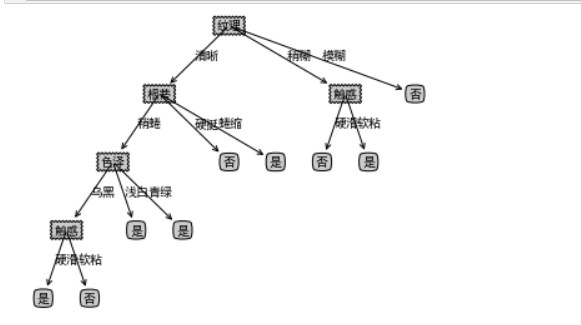• 决策树的剪枝

## 1.ID3代码

``````# 读取西瓜数据集
import numpy as np
import pandas as pd
# 由于上面的数据中包含了中文汉字，所以需要对数据进一步处理
'''

'''
df['色泽']=df['色泽'].map({'浅白':1,'青绿':2,'乌黑':3})
df['根蒂']=df['根蒂'].map({'稍蜷':1,'蜷缩':2,'硬挺':3})
df['敲声']=df['敲声'].map({'清脆':1,'浊响':2,'沉闷':3})
df['纹理']=df['纹理'].map({'清晰':1,'稍糊':2,'模糊':3})
df['脐部']=df['脐部'].map({'平坦':1,'稍凹':2,'凹陷':3})
df['触感'] = np.where(df['触感']=="硬滑",1,2)
df['好瓜'] = np.where(df['好瓜']=="是",1,0)
#由于西瓜数据集样本比较少，所以不划分数据集，将所有的西瓜数据用来训练模型
Xtrain = df.iloc[:,:-1]
Xtrain = np.array(Xtrain)
Ytrain = df.iloc[:,-1]
# 调用sklearn内置的决策树的库和画图工具
from sklearn import tree
import graphviz
# 采用ID3算法，利用信息熵构建决策树模型
clf = tree.DecisionTreeClassifier(criterion="entropy")
clf = clf.fit(Xtrain,Ytrain)
# 绘制决策树的图形
feature_names = ["色泽","根蒂","敲声","纹理","脐部","触感"]
dot_data = tree.export_graphviz(clf
,feature_names=feature_names
,class_names=["好瓜","坏瓜"]
,filled=True
,rounded=True
)
graph = graphviz.Source(dot_data)
graph
```1234567891011121314151617181920212223242526272829303132333435363738394041```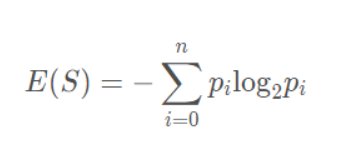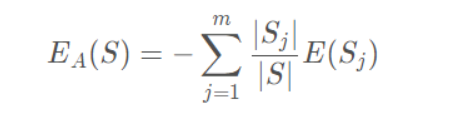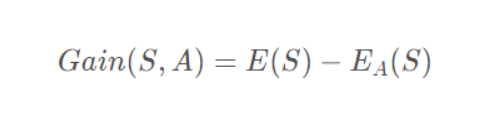ID3中样本分布越均匀，它的信息熵就越大，所以其原则就是样本熵越小越好，也就是信息增益越大越好。

## 2.C4.5实现

（1）对比ID3的改进点
C4.5算法是用于生成决策树的一种经典算法，是ID3算法的一种延伸和优化。C4.5算法对ID3算法进行了改进 ，改进点主要有：

（2）特征选择

（3）信息增益率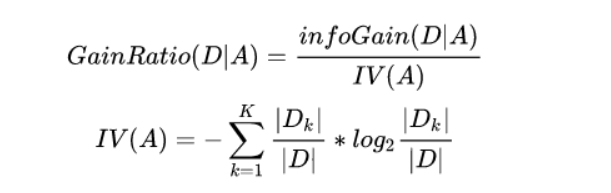（4）对连续特征的处理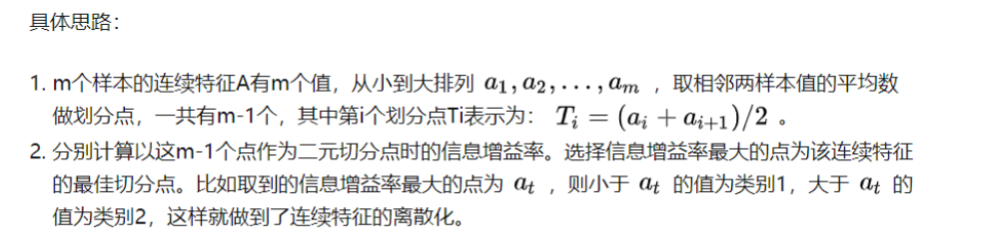（5）剪枝

## 3.CART算法

CART算法构造的是二叉决策树，决策树构造出来后同样需要剪枝，才能更好的应用于未知数据的分类。CART算法在构造决策树时通过基尼系数来进行特征选择。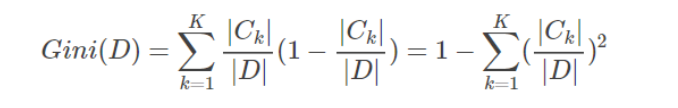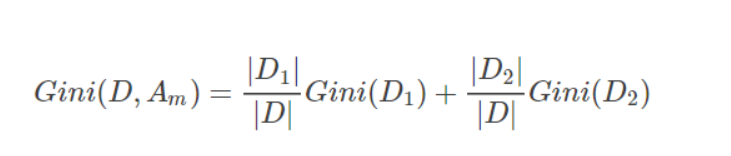• 输入： 训练数据集D，特征集A，结点样本数阈值δ，基尼指数阈值ϵ
• 输出： CART二叉决策树TT
• step1 若样本特征集为空，则TT是一棵单节点数，其类别为D中样本数最多的类，返回T；否则，转到step2。
• step2
对数据集D，计算特征集A中所有特征所有可能切分点的基尼指数，若基尼指数的值均小于给定阈值ϵ，则TT是一棵单节点数，其类别为D中样本数最多的类，返回T；否则，转到step3。
• step3
选择基尼指数最小的特征Amin和相应切分点α作为根节点的特征值和切分标准，根据D中样本是否等于α（或≤α≤α，或≥α≥α）将D分为两个子集D1和D2，将D1和D2分别分配到两个子结点中，若子结点样本数均小于给定阈值δ，则该子结点是一个叶结点，若两个子结点均为叶结点，返回T；否则，返回T并转到step4。
• step4 对于非叶子结点，令D等于该子结点所对应的数据集，特征集A=A−{Amin}，递归的调用step1-step3，返回子树T。
• 输入： CART算法生成的决策树T
• 输出： 最优决策树Tα
• step1 若T为单结点树或由一个根结点和两个叶结点构成的树，返回T；否则，k=0,α0=0,T0=T，转到step2。
• step2
k=k+1，计算Tk−1所有内部结点t的g(t)值，g(t)的最小值为αk，g(t)取最小值处的结点为tk，剪掉以tk为根结点的子树Ttk，并以tk处类别最多的样本作为新的叶结点tk的类，得到[αk,αk+1)上的最优子树Tk。
• step3 若Tk是由一个根结点和两个叶结点构成的树，转到step4；否则，转到step2。
• step4
利用验证数据集对最优子树序列T0,T1,⋯,TL进行测试，选择对于验证数据集有最小基尼指数的最优子树作为最终的最优决策树Tα，返回Tα。